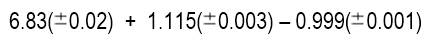# Problem: Find the absolute uncertainty for the following calculation.

###### Problem Details

Find the absolute uncertainty for the following calculation.Frequently Asked Questions

What scientific concept do you need to know in order to solve this problem?

Our tutors have indicated that to solve this problem you will need to apply the Uncertainty concept. You can view video lessons to learn Uncertainty. Or if you need more Uncertainty practice, you can also practice Uncertainty practice problems.

What is the difficulty of this problem?

Our tutors rated the difficulty ofFind the absolute uncertainty for the following calculation....as medium difficulty.

How long does this problem take to solve?

Our expert Analytical Chemistry tutor, Jules took 1 minute and 16 seconds to solve this problem. You can follow their steps in the video explanation above.